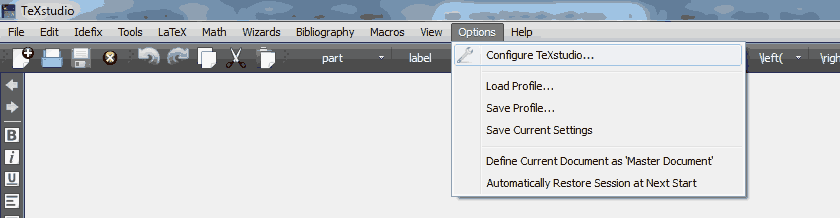# Mathematics CIIT: LaTeX ResourcesThis page contains LaTeX template of CIIT Mathematics, MSc Project and MS Thesis templates.

Download a zip file given below and extract it by right clicking on the file.

The latest templates are available at http://www.mathcity.org/cui

Three separte software and installation are needed to run the LaTeX on windows.

After installing above software, configure Sumatra PDF and TeXstudio for forward and inverse search.

To activate forward and inverse search, go in TeXstudio and Option > Configure TeXstudio > Commands > External PDF viewerReplace it with following code:

For 32bit Operating System

"C:\PROGRA~1\SumatraPDF\SumatraPDF.exe" -reuse-instance -forward-search "?c:am.tex" @ -inverse-search """""C:\PROGRA~1\TeXstudio\texstudio.exe"""" """%%f""" -line %%l" "?am.pdf"

For 64bit Operating System (if 64bit SumatraPDF is installed)

"C:\PROGRA~1\SumatraPDF\SumatraPDF.exe" -reuse-instance -forward-search "?c:am.tex" @ -inverse-search """""C:\PROGRA~2\TeXstudio\texstudio.exe"""" """%%f""" -line %%l" "?am.pdf"

For 64bit Operating System (if 32bit SumatraPDF is installed)

"C:\PROGRA~2\SumatraPDF\SumatraPDF.exe" -reuse-instance -forward-search "?c:am.tex" @ -inverse-search """""C:\PROGRA~2\TeXstudio\texstudio.exe"""" """%%f""" -line %%l" "?am.pdf"

In above code, we considered that windows in installed in C drive. If it is installed in different drive then, please use the respective code instead of C. Also it was considered that programs are installed on their default location.

In the Build select “PDF Viewer: External PDF Viewer”

### LaTeX Codes

• To write inline equation

Use a $\$ $(dollar) sign to write equation or symbols in between statements or sentences, e.g. Let$I$be an interval in$\mathbb{R}$and$f:I\to \mathbb{R}$be a function Let$I$be an interval in$\mathbb{R}$and$f:I\to \mathbb{R}$be a function • To write an equation Use double dollar$(\$\$)\$ to write dedicated equation, e.g.,
$$\sin^2 \theta + \cos^2 \theta =1$$

$$\sin^2 \theta + \cos^2 \theta =1$$

If you wish that equation number appear automatically to this equation, then write in the following way:

\begin{equation}
\sin^2 \theta + \cos^2 \theta =1
\end{equation}

\begin{equation} \sin^2 \theta + \cos^2 \theta =1 \end{equation}

For more tips and tricks related to TeXStudio, please visit: http://www.texstudio.org/#features

• ciit
• Last modified: 9 months ago
• by Administrator